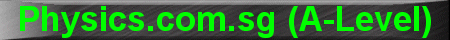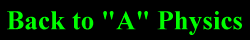(New tips are continually added to these pages.  Check back in a few months' time for more)

TOPIC 1:    Units, Dimensions, Errors and Uncertainties

Tip 1:

What is the difference between Errors and Uncertainties?  Errors are uni-directional whereas Uncertainties are bi-directional.  Therefore, Errors can be added or subtracted, but Uncertainties can only be added.  (Note: this statement may sound paradoxical but the following example shall illustrate).

For example, let's use the formula for the period of pendulum:

T = 2p Ö(l/g)

Error

The length of the pendulum, l, which is supposed to be 0.80 m, is measured as 0.86 m (with an error of +0.06m).  The acceleration due to gravity, g, which is supposed to be 9.81 m s-2, is measured as 9.84 m s-2 (with an error of +0.03 m s-2)

To find the error that results in the measurement of the period T, we have to first find the fractional error of l and g.

Dl / l = 0.06 / 0.80 = 0.075

Dg / g = 0.03 / 9.81 = 0.003

Since l is in the numerator, its positive error will result in an increase in the period T.  Since g is in the denominator, its positive error will result in a decrease in the period T.  Therefore, the fractional error of l/g will be 0.075 - 0.003 = 0.072

Since l/g is square-rooted (ie. to the power of 1/2), the fractional error of of Dl/g will be 1/2 x 0.072 = 0.036.

Next, evaluate the period T by substituting l = 0.80m and g = 9.81 m s-2 , we find that T = 1.794s

The error of T will be its value (1.794 s) multiplied by the fractional error (0.036), the answer is 0.065, or 0.07 after rounding off to 1 significant figure.  The erroneous value is therefore 1.79 + 0.07 = 1.86 s.

Let's check the answer by substituting l = 0.86m and g = 9.84 m s-2 into the equation, the answer is 1.86 s.

Uncertainty

Using the same example, instead of a positive error of +0.06 m for the length l, we have an uncertainty of +0.06 m; and instead of a positive error of +0.03 m s-2 for the acceleration due to gravity g, we have an uncertainty of +0.03 m s-2.  Then the total fractional uncertainty is 0.075 + 0.003 = 0.078.

The uncertainty of T will be its value (1.794) multiplied by the fractional uncertainty (0.078), the answer is 0.14.

Therefore, period T = 1.794 + 0.14, but since uncertainties have to be rounded upward to only have 1 significant figure, the proper presentation is T = (1.8 + 0.2) s.